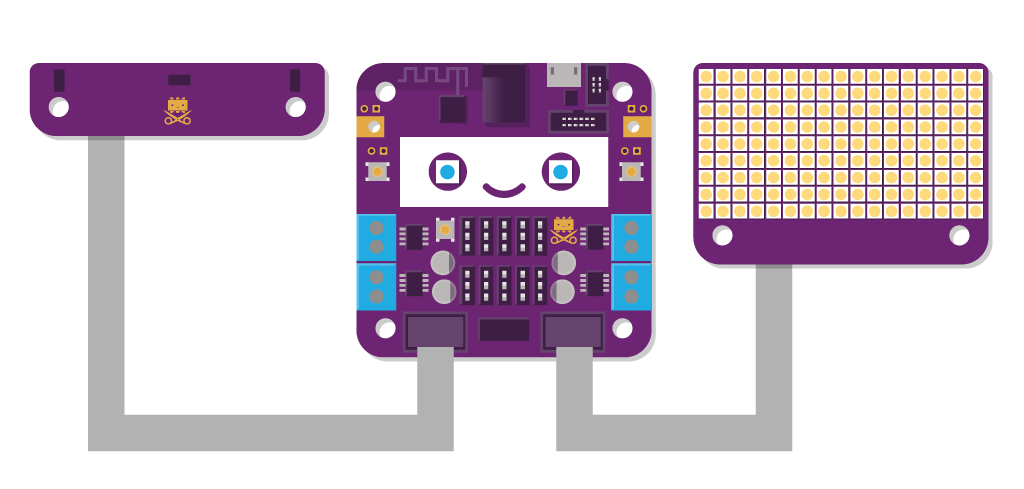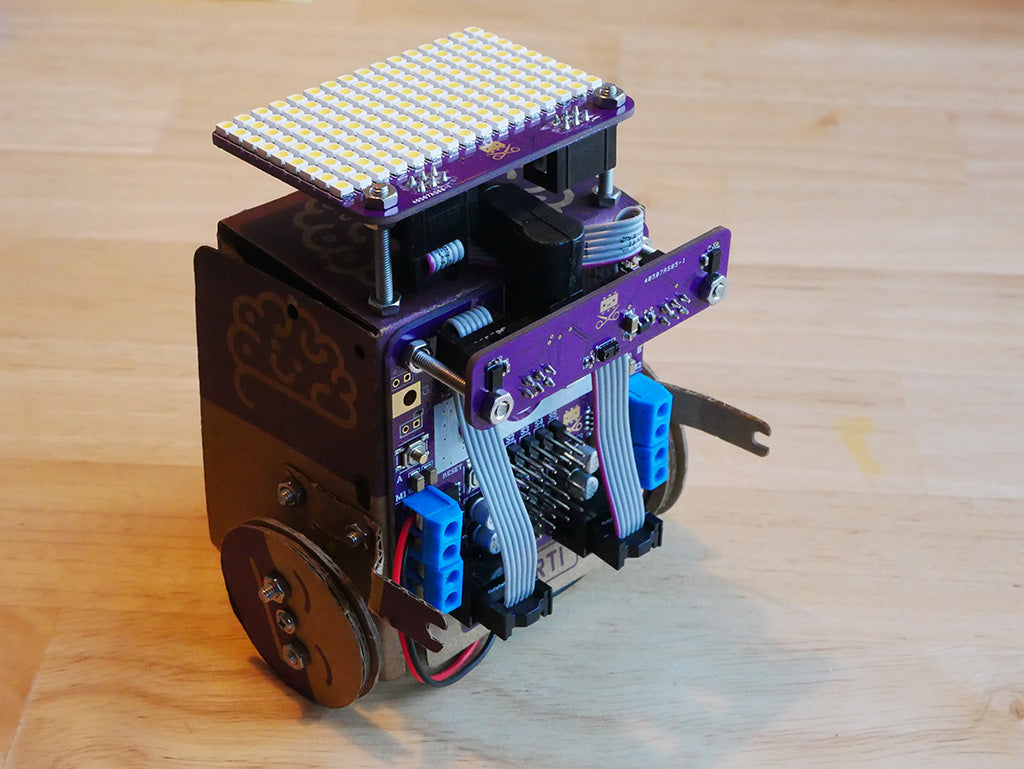# Wall avoiding robot with Distance Sensors and L.E.D. MatrixIn this tutorial we will use the two extra circuit boards in the Genius Kit to make Smartibot drive on it's own and avoid obstacles. We will use the the two distance sensors to check if there is an obstacle in front of Smartibot and which would be the best way to turn and we will use the L.E.D. matrix, mounted on top of Smartibot to graph the distance readings from each distance sensor, so it is easier to see what is going on.

## Physical Set-up

The first thing we need to do is connect up the extra circuit boards and attach them to Smartibot. This is easiest to do on the A.I. Bot.Swap the short bolts holding on the main circuit board at the top for some long ones, put nuts on to hold the main circuit board and then two more about 5mm from the end of each bolt. Plug one end of the small flat cable into E1 and the other into the socket on the left side of the distance/gesture board. Slide the board over the long bolts and put on two more nuts. If you tighten the nuts on each side of the distance/gesture board you should be able to get them to grip it nicely. Use two more long bolts some more nuts to hold the L.E.D. matrix on top of Smartibot and connect the left hand socket on this to E2 using the longer flat cable.

## Code

First of all make sure that you have the latest firmware installed on your Smartibot, follow the instructions here to update.

We need to include three libraries - the one for Smartibot, and the ones for the L.E.D. Matrix and the distance/gesture board:

```var smarti = require("Smartibot"); ```

```var dist = require("Smartibot-distance").connect(smarti.E1); ```

`var g = require("Smartibot-display").connect(smarti.E2);`

Next we need to create some variables. Two of these are used to smooth out the readings from the distance sensors as they can be a bit noisy and if we don't smooth them we get some strange Smartibot behaviour. The other variable is to control the time interval at which the program repeats. We want a longer one for when Smartibot is going straight to when it is turning:

`var right = 300; ``//smoothed out value for right sensor distance```` ```

`var left = 300; ``//smoothed out value for left sensor distance```` ```

`var int = 100; ``//Time interval between repeats of the control process`

Now we need to create a block of code that runs over and over again (to read the distance values and draw the graphs on the display and control the motors). We can do this by creating a loop like this:

`setInterval(function() {`

`}, int);`

Inside this loop we will put all the code we want to run over and over again. The first part just smooths out the readings from the distance sensors slightly by taking the average of the latest reading and the previous one:

`right = (right + dist.getRight())/2; `

`left = (left + dist.getLeft())/2;`

The next bit does everything we need to visualise the distance sensor outputs as lines on each side of the LED Matrix.

`g.clear(); ``//Clear the LED Matrix```` ```

`g.setColor(127); ``// set brightness to 50% (range is 0 to 255)```` ```

`// Draw rectangles based on values from distance sensors`` `

```g.drawRect(0,0,0,(left/100)); ```

```g.drawRect(15,0,15,(right/100)); ```

```// Send what we drew to the LED Matrix ```

`g.flip();`

Next we need to put in a sequence of blocks of code to make Smartibot do different things depending on how far away from an obstacle it is. The first one makes Smartibot drive backwards if both distance sensors are less than 10cm from an obstacle:

``` // If obstacle is less than 10cm from both sensors drive backwards ```

``` if (right < 100 && left < 100){ ```

``` smarti.setMotor(1,-0.7); ```

``` smarti.setMotor(2,0.7); ```

` }`

The next section makes Smartibot turn towards the sensor that is further from the obstacle if both sensors are less than 20cm from an obstacle:

``` //If obstacle is less than 20cm from both sensors turn towards the sensor that is further from the obstacle ```

``` else if(right < 200 && left < 200){ ```

``` if(right > left){ ```

``` smarti.setMotor(1,0.7); ```

``` smarti.setMotor(2,0.7); ```

``` int = 100; } ```

``` else{ ```

``` smarti.setMotor(1,-0.7); ```

``` smarti.setMotor(2,-0.7); ```

``` int = 100; } ```

` }`

The last section of code makes Smartibot drive forward if the distance sensors are more than 20cm away from the obstacle (so none of the conditions above are met):

``` else{ ```

``` smarti.setMotor(1,0.7); ```

``` smarti.setMotor(2,-0.7); ```

` int = 200; ``}`

That is it!

Here all of the code together so you can just drop it into Espruino and load it onto your Smartibot:

```var smarti = require("Smartibot"); ```

```var dist = require("Smartibot-distance").connect(smarti.E1); ```

```var g = require("Smartibot-display").connect(smarti.E2); ```

`var ````int = 100; ```

```var right = 300; ```

`var left = 300;`

`//If you want to save this code onto your Smartibot to run whenever you switch it uncomment out the line below and the final one. `

`// E.on('init', function() {`

```setInterval(function() { ```

```//Smooth out values from distance sensors to reduce noise ```

```right = (right + dist.getRight())/2; ```

```left = (left + dist.getLeft())/2; ```

``` g.clear(); //Clear the LED Matrix ```

`g.setColor(127); `

```// set brightness to 50% (range is 0 to 255) ```

`// Draw rectangles based on values from distance sensors `

```g.drawRect(0,0,0,(left/100)); ```

```g.drawRect(15,0,15,(right/100)); ```

```// Send what we drew to the LED Matrix ```

```g.flip(); ```

``` // If obstacle is less than 10cm from both sensors drive backwards ```

``` if (right < 100 && left < 100){ ```

``` smarti.setMotor(1,-0.7); ```

``` smarti.setMotor(2,0.7); } ```

` ``//If obstacle is less than 20cm from both sensors turn towards the sensor that is further from the obstacle```` ```

``` else if(right < 200 && left < 200){ ```

``` if(right > left){ ```

` smarti.setMotor(1,0.7);`

``` smarti.setMotor(2,0.7); ```

` int = 100; `}

``` else{ ```

``` smarti.setMotor(1,-0.7); ```

``` smarti.setMotor(2,-0.7); ```

``` int = 100; } ```

``` } ```

``` //Otherwise drive forward in a straight line ```

``` else{ ```

``` smarti.setMotor(1,0.7); ```

``` smarti.setMotor(2,-0.7); ```

``` int = 200; } ```

`}, int);`

`// });`